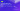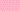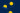Pricing# What is a growing perpetuity?

If you want to find out the present value of your company’s cash flows, the growing perpetuity equation could be a useful tool. But what is a growing perpetuity? Explore everything you need to know with our comprehensive guide.

## Understanding growing perpetuities

Perpetuities are securities or cash flows that pay out for an infinite amount of time. A growing perpetuity is a cash flow that is not only expected to be received ad infinitum, but also grow at the same rate of growth forever. For example, if your business has an investment that you expect to pay out £1,000 forever, this investment would be considered a perpetuity. However, if you expect to receive £1,000 in the first year, and for the investment to grow at a rate of 5% in perpetuity, it would be considered a growing perpetuity.

## Examples of a growing perpetuity

There are many different examples of a growing perpetuity that you may encounter in your everyday life. Stock valuations, for example, always assume a growing perpetuity. At the same time, commercial real estate companies may use a growing perpetuity formula when calculating the future value of their rental cash flows, which may be considered indefinite. So, if you’re investing in anything based on perpetuity, growing perpetuity equations could be helpful.

## How to calculate the present value of a growing perpetuity

The present value of a growing perpetuity is a way to understand the current value of an infinite series of cash flows growing at a steady rate, ad infinitum. Although the total value of the cash flows is infinite, the present value is finite. Due to the time value of money concept, the further the cash flows are into the future, the lower their present value will be. You can use the following growing perpetuity formula to calculate the present value of a growing perpetuity:

Present Value of a Growing Perpetuity = Year 1 Cash Flow / (Discount Rate – Growth Rate)

So, how does this work in practice? Let’s take a look at an example of a growing perpetuity. Imagine that Company A purchased several stocks with an annual cash flow of £1,500 that is expected to continue indefinitely. Moreover, the cash flow is expected to grow at a rate of 7% each year, and the required return on investment (used for the discount rate) is 12%. Using the growing perpetuity formula above, we can calculate the present value of the growing perpetuity like so:

Present Value of a Growing Perpetuity = £1,500 / (0.12 – 0.07) = £30,000

This means that the present value of Company A’s cash flow is £30,000. If this figure is higher than the amount Company A paid for the stocks, it was likely to have been a smart investment.

## How to calculate the future value of a growing perpetuity

To work out the future value of a growing perpetuity, you’ll need a future date. However, this effectively transforms the growing perpetuity into an annuity (a fixed cash flow that’s received for a specific amount of time). As such, this metric isn’t as useful as a growing perpetuity equation. If you do want to work out the future value of a growing perpetuity, you’ll need to use the net present value (NPV) formula.

## We can help

GoCardless helps you automate payment collection, cutting down on the amount of admin your team needs to deal with when chasing invoices. Find out how GoCardless can help you with ad hoc payments or recurring payments.

Interested in automating the way you get paid? GoCardless can help

Contact sales